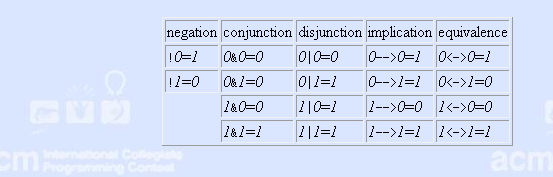# 1442. Boolean Logic

Propositions are logical formulas consisting of proposition symbols and connecting operators. They are recursively defined by the following rules:

1.All proposition symbols (in this problem, lower-case alphabetic characters, e.g., a and z) are propositions.

2.If P is a proposition, (!P) is a proposition, and P is a direct subformula of it.

3.If P and Q are propositions, (P&Q), (P|Q), (P–>Q), and (P<->Q) are propositions, and P and Q are direct subformulas of them.

4.Nothing else is a proposition.

The operations !, &, |, –>, and <-> denote logical negation, conjunction, disjunction, implication, and equivalence, respectively. A proposition P is a subformula of a proposition R if P=R or P is a direct subformula of a proposition Q and Q is a subformula of R.

Let P be a proposition and assign boolean values (i.e., 0 or 1) to all proposition symbols that occur in P. This induces a boolean value to all subformulas of P according to the standard semantics of the logical operators:This way, a value for P can be calculated. This value depends on the choice of the assignment of boolean values to the proposition symbols. If P contains n different proposition symbols, there are 2n different assignments. To evaluate all possible assignments we may use truth tables.

A truth table contains one line per assignment (i.e., 2n lines in total). Every line contains the values of all subformulas under the chosen assignment. The value of a subformula is aligned with the proposition symbol, if the subformula is a proposition symbol, and with the center of the operator otherwise.

### 输入格式

The input contains several test cases, each on a separate line. Every test case denotes a proposition and may contain arbitrary amounts of spaces in between. The input file terminates immediately after the newline symbol following the last test case.

### 输出格式

For each test case generate a truth table for the denoted proposition. Start the truth table by repeating the input line. Evaluate the proposition (and its subformulas) for all assignments to its variables, and output one line for each assignment. The line must have the same length as the corresponding input line and must consist only of spaces and the characters 0 and 1. Output an empty line after each test case.

Let s1,…,sn be the proposition symbols in the denoted proposition sorted in alphabetic order. Then, all assignments of 0 to s1 must precede the assignments of 1 to s1. Within each of these blocks of assignments, all assignments of 0 to s2 must precede the assignments of 1 to s2, and so on.

1 人解决，4 人已尝试。

1 份提交通过，共有 5 份提交。

9.9 EMB 奖励。